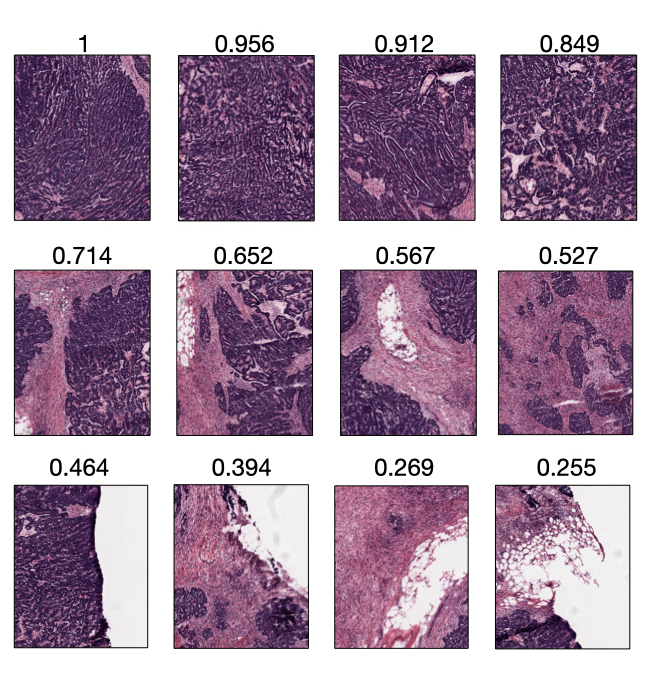# Scorer¶

The goal of the scorer module is to provide the grading functions for the ScoreTiler extractor. The scorer objects input a Tile object and return their computed score.Example of tiles scored by the NucleiScorer object with their respective score scaled between 0 and 1. Tiles are extracted from the ovarian tissue WSI.¶
class CellularityScorer(*args, **kwds)[source]

Implement a Scorer that estimates the cellularity in an H&E-stained tile.

This class deconvolves the hematoxylin channel and uses the fraction of tile occupied by hematoxylin as the cellularity score.

Notice that this scorer is useful when tiles are extracted at a very low resolution with no artifacts; in this case, using theNucleiScorer() instead would not work well as nuclei are no discernible at low magnification.

__call__(tile)[source]

Return the tile cellularity score.

Parameters
• tile (Tile) – The tile to calculate the score from.

• consider_tissue (bool) – Whether the cellularity score should be computed by considering the tissue on the tile. Default is True

Returns

Cellularity score

Return type

float

Parameters

consider_tissue (bool, optional) – Whether the detected tissue on the tile should be considered to compute the cellularity score. Default is True

Notes

If the tile presents artifacts (e.g., tissue folds, markers), the scorer cannot be fully trusted.

class NucleiScorer(*args, **kwds)[source]

Implement a Scorer that estimates the presence of nuclei in an H&E-stained tile.

This class implements an hybrid algorithm that combines thresholding and morphological operations to segment nuclei on H&E-stained histological images.

The NucleiScorer class defines the score of a given tile t as:

$s_t = N_t\cdot \mathrm{tanh}(T_t) \mathrm{, } \; 0\le s_t<1$

where $$N_t$$ is the nuclei ratio on t, computed as number of white pixels on the segmented mask over the tile size, and $$T_t$$ the fraction of tissue in t.

Notice that we introduced the hyperbolic tangent to bound the weight of the tissue ratio over the nuclei ratio.

Notes

If the tile presents artifacts (e.g., tissue folds, markers), the scorer cannot be fully trusted.

__call__(tile)[source]

Return the nuclei score associated with the tile.

Parameters

tile (Tile) – The tile to calculate the score from.

Returns

Nuclei score

Return type

float

class RandomScorer(*args, **kwds)[source]

Implement a Scorer that returns a random float score between 0 and 1.

__call__(tile)[source]

Return the random score associated with the tile.

Parameters

tile (Tile) – The tile to calculate the score from.

Returns

Random score ranging between 0 and 1.

Return type

float

class Scorer(*args, **kwds)[source]

General scorer object

abstract __call__(tile)[source]

Call self as a function.

Parameters

tile (histolab.tile.Tile) –

Return type

float